# Tricky Mathematical Concepts Easily Explained In 21 Brilliant GIFs

Math is tough, that’s a fact. Math has been the “downfall” of many students – that’s another fact. There is simply no getting around learning it – or so we thought.

Check out these 21 cool GIFs that can help you visualize some tricky aspects of math. Bet you’ll ace your next exams with these.

1. “If an arc of a circle is the same length as its radius, the resulting angle is one radian:”via Wikimedia Commons

2. “Visualizing sine (red) on the Y axis and cosine (blue) on the X axis. The relative position of the circle is shown in black:”via imgur

3. “This shows the same thing, but a bit more simply:”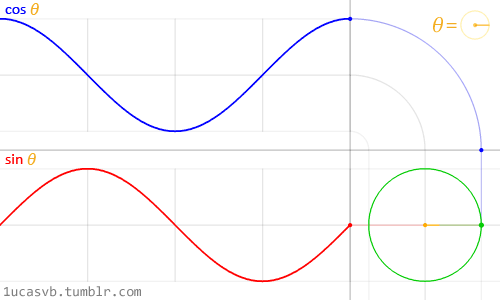via Reddit

4. “Here’s how sine and cosine apply to triangles:”via imgur

5. “Cosine is the derivative of sine:”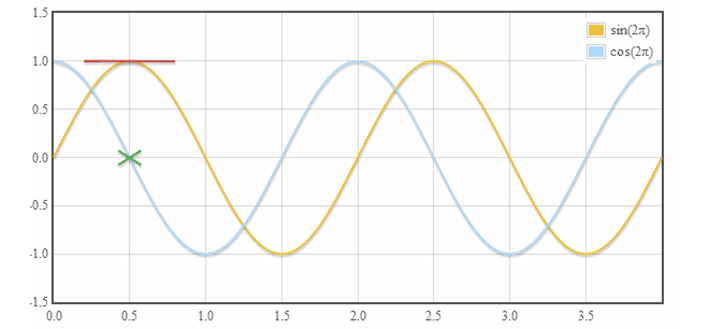via Wikimedia Reddit

6. “Converting a function from Cartesian to Polar coordinates:”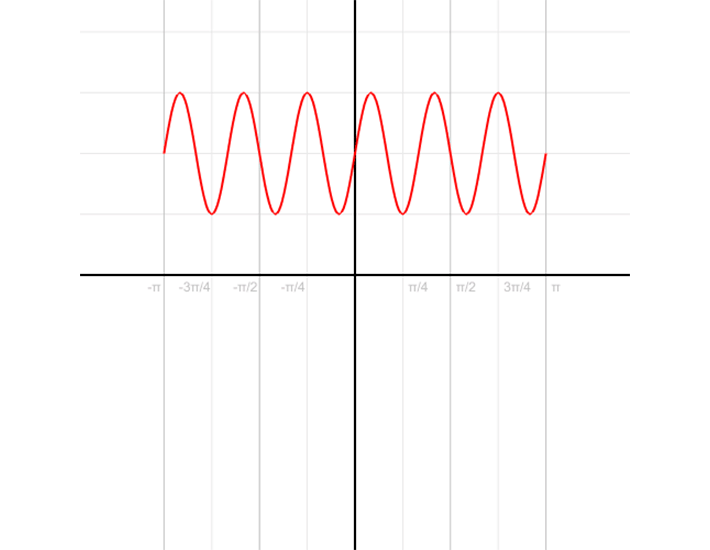via Wikimedia Commons

7. How to draw a parabolavia giphy

8. “Use FOIL to easily multiply binomials:”via mathcaptain

9. How to create an ellipsevia giphy

10. Hyperbolavia giphy

11. “Translating that into 3D, you get a hyperboloid. Believe it or not, it’s made with completely straight lines:”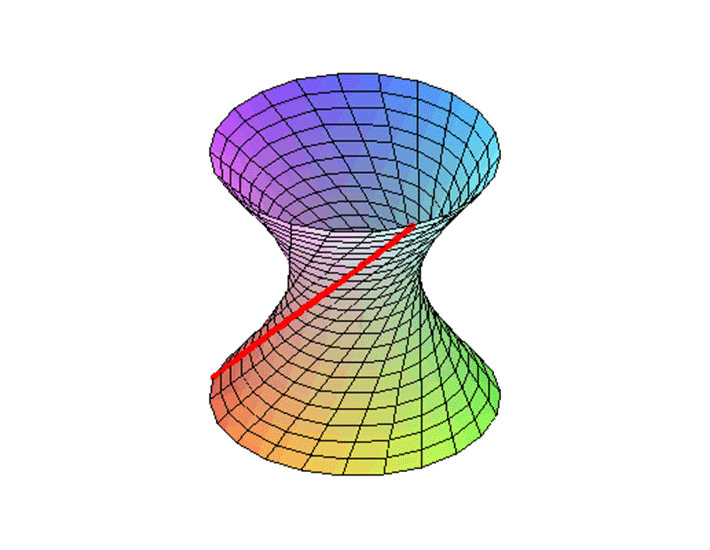via tumblr

12. You can even make it do this: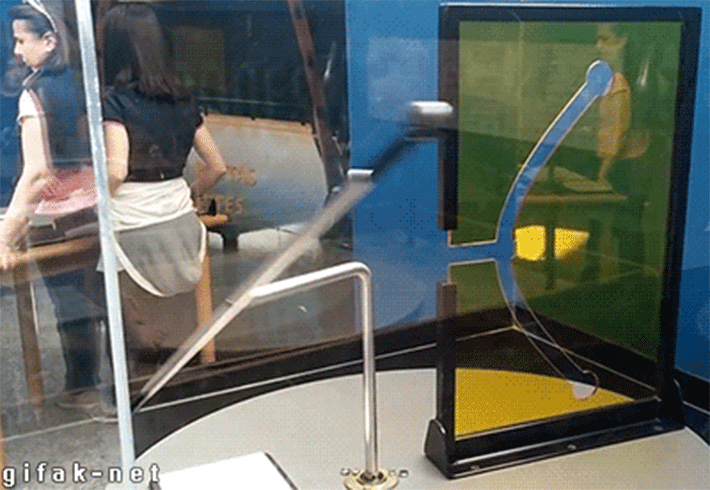via tumblr

13. “Use this trick so you don’t get mixed up when doing matrix transpositions:”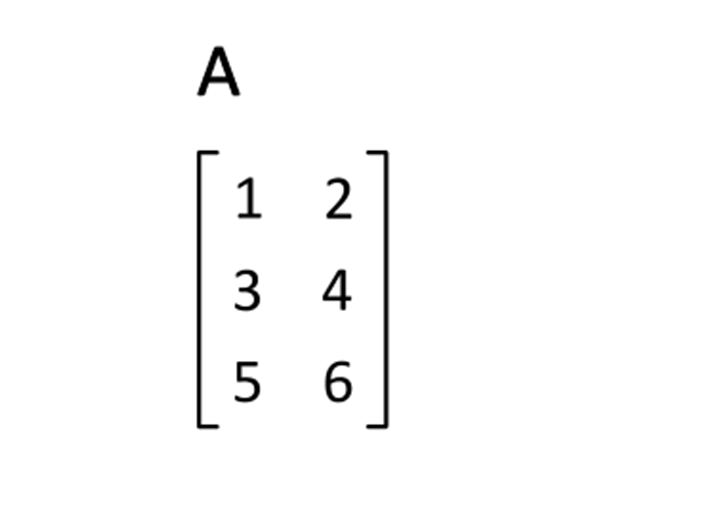via Wikimedia Commons

14. “If you’re studying trig, you better get pretty comfortable with circles. Check out this visualization that shows what you’re really looking at when you deal with pi:”via imgur

15. “Exterior angles of polygons will ALWAYS add up to 360 degrees:”via math.stackexchange

16. “What the Pythagorean Theorem is really trying to show you:”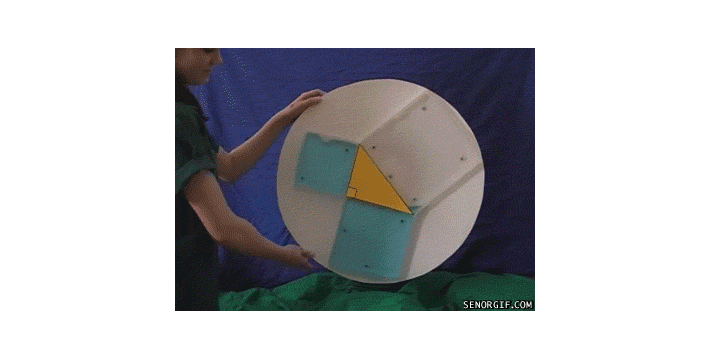via giphy

17. “The Riemann sum is the approximate area under a curve:”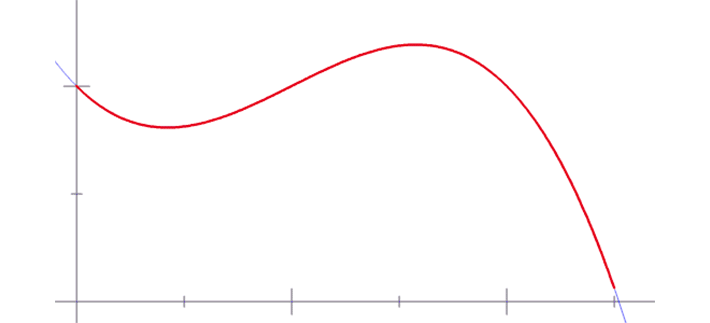via giphy

18. How to solve logarithms:via imgur

19. Tangent lines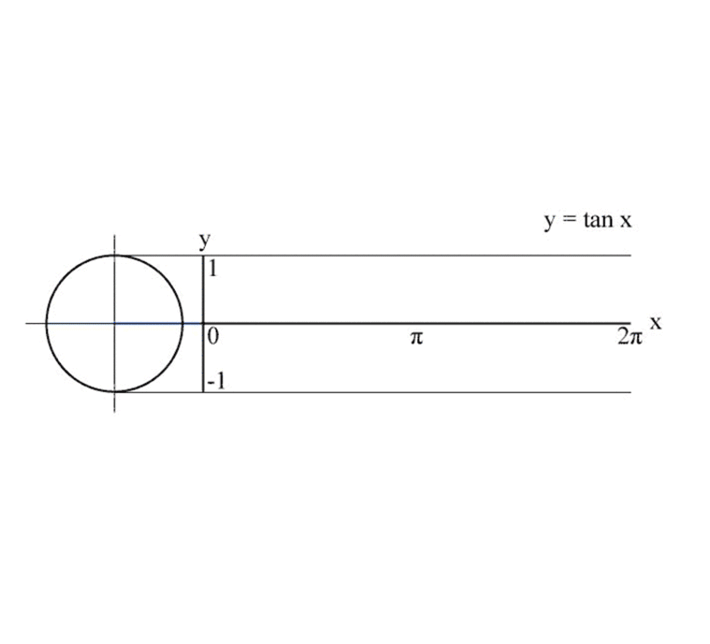via John Reid │ Wikimedia Commons

20. “Flipped on its side, the shape begins to make more sense:”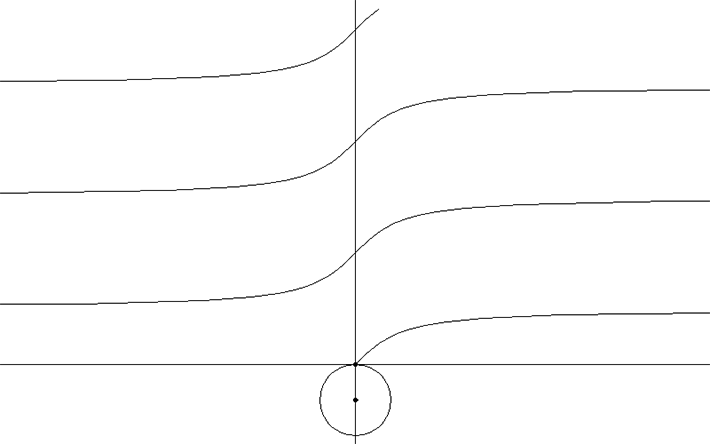via imgur

21. “Solving pascal triangles”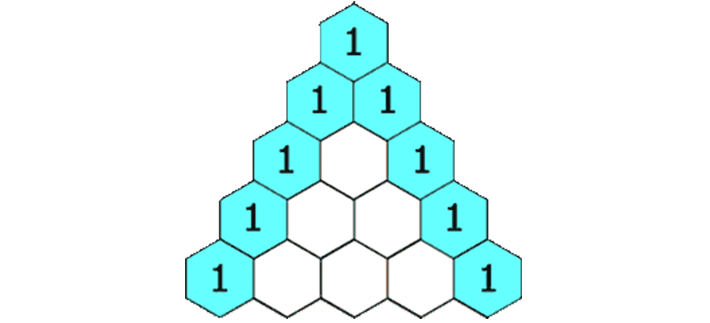via Hersfold │ Wikimedia Commons